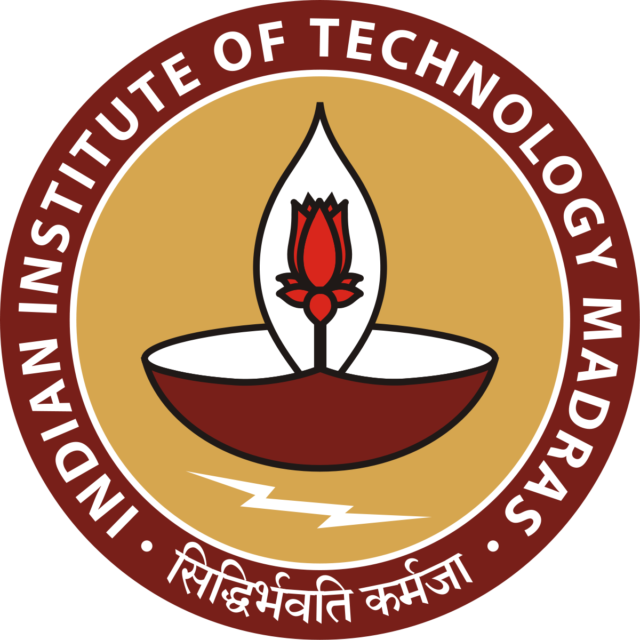Home Certification IIT Madras Data Science 8 Weeks Free Course for Students | IIT...

# IIT Madras Data Science 8 Weeks Free Course for Students | IIT Certificate -Register Now

0# IIT Madras Data Science 8 Weeks Course for Students | IIT Certificate -Register Now

## HIGHLIGHTS :

Learning Objectives :

• Introduce R as a programming language
• Introduce the mathematical foundations required for data science
• Introduce the first level data science algorithms
• Introduce a data analytics problem solving framework
• Introduce a practical capstone case study

Learning Outcomes:

• Describe a flow process for data science problems (Remembering)
• Classify data science problems into standard typology (Comprehension)
• Develop R codes for data science solutions (Application)
• Correlate results to the solution approach followed (Analysis)
• Assess the solution approach (Evaluation)
• Construct use cases to validate approach and identify modifications required (Creating)

### Course layout

Week 1:  Course philosophy and introduction to R

Week 2:  Linear algebra for data science

• Algebraic view – vectors, matrices, product of matrix & vector, rank, null space, solution of over-determined set of equations and pseudo-inverse)
• Geometric view – vectors, distance, projections, eigenvalue decomposition

Week 3:  Statistics (descriptive statistics, notion of probability, distributions, mean, variance, covariance, covariance matrix, understanding univariate and multivariate normal distributions, introduction to hypothesis testing, confidence interval for estimates)

Week 4:  Optimization

Week 5:  1. Optimization 2. Typology of data science problems and a solution framework

Week 6:  1. Simple linear regression and verifying assumptions used in linear regression  2. Multivariate linear regression, model assessment, assessing importance of different variables, subset selection

Week 7:  Classification using logistic regression

Week 8:  Classification using kNN and k-means clustering

### Course certificate :

The course is free to enroll and learn from. But if you want a certificate, you have to register and write the proctored exam conducted by us in person at any of the designated exam centres.
The exam is optional for a fee of Rs 1000/- (Rupees one thousand only).
Date and Time of Exams: 27 March 2022